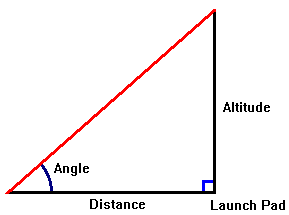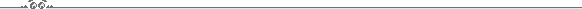Calculating a Rockets Altitude

 There are three ways you can calculate rocket altitude ... performance, prediction and recording. Performance requires a device to measure the angle the rocket reaches from a known distance from the launch pad (most accurate if you can keep the angle below 45 degrees) and a mathematical formula. Making the calculations will require a calculator that has trigonometric functions (sin, cos, tan). The formula is (Tangent of angle) x (Distance from launch pad) = Rocket AltitudeExample 1: If you are one hundred yards (300 feet) away from the pad, and you measure an angle of 45 degrees, the tangent of 45 = 1 1 x 300 = 300 feet altitude Example 2: If you are five hundred yards away from the pad, and you measure an angle of 39 degrees, the tangent of 39 = 0.810 0.810 x 500 yards = 405 yards = 1,215 feet altitude Example 3: This will illustrate the difference with errors above 45 degrees.  If you are five hundred feet away from the pad, and you measure an angle between 73 and 74 degrees, the tangent of 73 = 3.27, and the tangent of 74 = 3.48 3.27 x 500 feet = 1,635 feet altitude 3.48 x 500 feet = 1,740 feet altitude Here you can see a difference of 105 feet in just one degree of difference.  Since the tangent of 90 degrees is indefinable (technically it's infinity), a one degree difference between 88 and 89 at the same 500 foot distance from the launch pad shows a 14,327 foot difference.  The numerical representation for tangent angles greater than 45 degrees goes up exponentially, which is why you get the most accuracy at angles less than 45 degrees.  Try and move at least as many feet away as you would expect the rocket to fly (of course, if you expect it to go up to 1,000 feet, this may be impractical).The Prediction method is an inexpensive (free) way to predict a rockets expected altitude using a computer program.  I use the altitude prediction program ALTPRED.  It is a zipped freeware Windows based program that has all the Estes motors built into it's database, and the zipped file includes the APD files for you to import high-power data.  All you need to do it input the diameter of the rocket, it's empty weight, and select a motor and it will give you all the details of the flight ... altitude, speed, etc.  Granted this is only a prediction program, and there are many variables that can affect the actual performance of the rocket but, personally, I don't need complete accuracy ... I don't work for NASA, and I can't tell the difference between a rocket that hits 1,200 feet versus one that hits 1,300 feet.The Recording method is the most accurate method, because you utilize on-board electronics to calculate the actual altitude that the rocket reaches.  Of course, adding electronics to your rockets adds a hefty expense and makes the design and construction more complicated, but the rewards are well worth it.  In addition to having the maximum altitude of the rocket recorded, there is also included electronics to automatically deploy your parachutes at pre-determined altitudes.  There are several excellent recording/chute deployment altimeters available through ADEPT Rocketry.  I use these electronics in conjunction with the ALTPRED program to verify the expected altitude.  Using actual recording data, I can tweak the prediction program to give me a more accurate prediction.